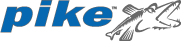# Pike

Appeared in:
1994
Influenced by:
Typing discipline:
File extensions:
.pike
Versions and implementations (Collapse all | Expand all):
Programming language

Pike is a dynamic interpreted language with a C-like syntax.

It was created in 1994 as a GPL version of LPC (which was not licensed for commercial use). Initially it was called µLPC (for micro LPC), but later was renamed for branding purposes.

Pike is an object-oriented language, with built-in garbage collection and a flexible system of data types. It is considered to be one of the fastest scripting languages existing.

## Elements of syntax:

Inline comments // /* ... */ yes varname = value; vartype varname; vartype varname = value; ( ... ) { ... } == != equal() !equal() < > <= >= == != returntype(subtype) f(type1 p1, type2 p2, ...) f(a,b,...) f() , if (condition){ ... } if (condition){ ... }else{ ... } while(1){ ... } while (condition){ ... } do{ ... } while (!condition); for (int i = 1; i <= 10; i++){ ... } for (int i = 10; i >= 1; i--){ ... }Pike logo

## Examples:

### Hello, World!:

Example for versions Pike 7.8
``````void main()
{
write("Hello, World!\n");
}
``````

### Fibonacci numbers:

Example for versions Pike 7.8

This example uses recursive definition of Fibonacci numbers.

``````int fibonacci(int n)
{
return ( n<=2 ? 1 : fibonacci(n-1) + fibonacci(n-2) );
}

void main()
{
for (int n=1; n<=16; n++)
write(fibonacci(n)+", ");
write("...\n");
}
``````

### Factorial:

Example for versions Pike 7.8

This example uses recursive factorial definition. Note that `int` data type is extended to bignum in case of overflow.

``````int factorial(int n)
{
return ( n<=1 ? 1 : n * factorial(n-1) );
}

void main()
{
for (int n=0; n<=16; n++)
write(n+"! = "+factorial(n)+"\n");
}
``````

Example for versions Pike 7.8
``````void main()
{
int A = (int)Stdio.stdin->gets();
if (A == 0)
{
return 0;
}
int B = (int)Stdio.stdin->gets();
int C = (int)Stdio.stdin->gets();
int D = (B*B-4*A*C);
write(D+"\n");
if (D == 0)
write(sprintf("x = %f\n",-B/2.0/A));
else if (D > 0)
{
write(sprintf("x1 = %f\n", (-B+sqrt(D))/2.0/A));
write(sprintf("x2 = %f\n", (-B-sqrt(D))/2.0/A));
}
else
{
write(sprintf("x1 = (%f, %f)\n", -B/2.0/A, sqrt(-D)/2.0/A));
write(sprintf("x1 = (%f, %f)\n", -B/2.0/A, -sqrt(-D)/2.0/A));
}
}
``````

### CamelCase:

Example for versions Pike 7.8

This example implements character-by-character string processing. The only thing to note is that Pike provides no data type for characters. `text[i]` would return an integer — ASCII-code of the corresponding character. To get i-th character as a string variable, one has to use `text[i..i]` which is operation of extracting substring from a string.

``````void main()
{
string text = lower_case(Stdio.stdin->gets());
string cc = "";
int i, lastSpace = 1;

for (i=0; i<sizeof(text); i++)
{
if (text[i] >= 'a' && text[i] <= 'z')
{
if (lastSpace == 1)
cc += upper_case(text[i..i]);
else
cc += text[i..i];
lastSpace = 0;
}
else
lastSpace = 1;
}
write(cc+"\n");
}
``````